Courses

# Test: Measures Of Central Tendency And Dispersion- 2

## 40 Questions MCQ Test Business Mathematics and Logical Reasoning & Statistics | Test: Measures Of Central Tendency And Dispersion- 2

Description
This mock test of Test: Measures Of Central Tendency And Dispersion- 2 for CA Foundation helps you for every CA Foundation entrance exam. This contains 40 Multiple Choice Questions for CA Foundation Test: Measures Of Central Tendency And Dispersion- 2 (mcq) to study with solutions a complete question bank. The solved questions answers in this Test: Measures Of Central Tendency And Dispersion- 2 quiz give you a good mix of easy questions and tough questions. CA Foundation students definitely take this Test: Measures Of Central Tendency And Dispersion- 2 exercise for a better result in the exam. You can find other Test: Measures Of Central Tendency And Dispersion- 2 extra questions, long questions & short questions for CA Foundation on EduRev as well by searching above.
QUESTION: 1

Solution:
QUESTION: 2

Solution:
QUESTION: 3

### What is the coefficient of mean deviation for the following distribution of height? Take deviation from AM.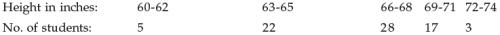Solution:
QUESTION: 4

The mean deviation of weight about median for the following data: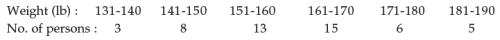Solution:
QUESTION: 5

What is the standard deviation from the following data relating to the age distribution of 200 persons?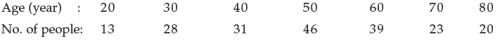Solution:
QUESTION: 6

What is the coefficient of variation for the following distribution of wages?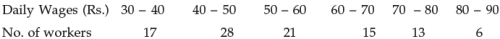Solution:
QUESTION: 7

Which of the following companies A and B is more consistent so far as the payment of dividend are concerned ?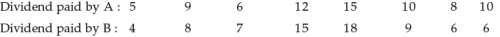Solution:
QUESTION: 8

The mean and SD for a group of 100 observations are 65 and 7.03 respectively. If 60 of these observations have mean and SD as 70 and 3 respectively, what is the SD for the group comprising 40 observations?

Solution:
QUESTION: 9

If two samples of sizes 30 and 20 have means as 55 and 60 and variances as 16 and 25 respectively, then what would be the SD of the combined sample of size 50?

Solution:
QUESTION: 10

The mean and SD of a sample of 100 observations were calculated as 40 and 5.1 respectively by a CA student who took one observation as 50 instead of 40 by mistake. The current value of SD would be

Solution:
QUESTION: 11

The value of appropriate measure of dispersion for the following distribution of daily wages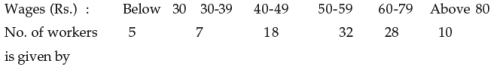Solution:
QUESTION: 12

​The no. of measures of central tendency is

Solution:
QUESTION: 13

The words “mean” or “average” only refer to

Solution:
QUESTION: 14

—————— is the most stable of all the measures of central tendency.

Solution:
QUESTION: 15

Mean is of ———— types.

Solution:
QUESTION: 16

Weighted A.M is related to

Solution:
QUESTION: 17

Frequencies are also called weights.

Solution:
QUESTION: 18

The algebraic sum of deviations of observations from their A.M is

Solution:
QUESTION: 19

G.M of a set of n observations is the ———— root of their product.

Solution:
QUESTION: 20

The algebraic sum of deviations of 8,1,6 from the A.M viz.5 is

Solution:
QUESTION: 21

G.M of 8, 4,2 is

Solution:
QUESTION: 22

——————— is the reciprocal of the A.M of reciprocal of observations.

Solution:
QUESTION: 23

A.M is never less than G.M

Solution:
QUESTION: 24

G.M is less than H.M

Solution:
QUESTION: 25

The value of the middlemost item when they are arranged in order of magnitude is called

Solution:
QUESTION: 26

Median is unaffected by extreme values.

Solution:
QUESTION: 27

Median of 2,5,8,4,9,6,71 is

Solution:
QUESTION: 28

The value which occurs with the maximum frequency is called

Solution:
QUESTION: 29

In the formula Mode = L1 + (d1 X c)/ (d1 + d2)

d1 is the difference of frequencies in the modal class & the —————— class.

Solution:
QUESTION: 30

In the formula Mode = L1 + (d1 X c)/ (d1 + d2)

d2 is the difference of frequencies in the modal class & the ———————— class.

Solution:
QUESTION: 31

In formula of median for grouped frequency distribution N is

Solution:
QUESTION: 32

When all observations occur with equal frequency ————— does not exit.

Solution:
QUESTION: 33

Mode of the observations 2,5,8,4,3,4,4,5,2,4,4 is

Solution:
QUESTION: 34

For the observations 5,3,6,3,5,10,7,2 , there are —————— modes.

Solution:
QUESTION: 35

—————— of a set of observations is defined to be their sum, divided by the no. of observations.

Solution:
QUESTION: 36

Simple average is sometimes called

Solution:
QUESTION: 37

When a frequency distribution is given, the frequencies of values are themselves treated as weights.

Solution:
QUESTION: 38

Each different value is considered only once for

Solution:
QUESTION: 39

Each value is considered as many times as it occurs for

Solution:
QUESTION: 40

Multiplying the values of the variable by the corresponding weights and then dividing the sum of products by the sum of weights is

Solution:

Track your progress, build streaks, highlight & save important lessons and more!

### Similar Content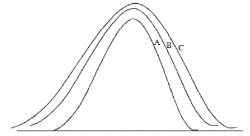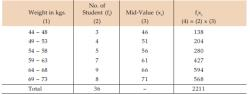### Related tests